The emission of radiation from an unstable nucleus is called its radioactivity. After the discovery of X rays in 1895 by William R\"ontgen, Henri Becquerel suspected that the glow produced by phosphorescent materials could be same as X rays. Phosphorescent materials glow after they have been exposed to light. To test his ideas, Becquerel placed several phosphorescent materials on photographic plates that were wrapped in black paper to shield them from direct sunlight. When he set them out in the sunlight he would find that the area beneath the phosphorescent materials would be darkened which seemed to confirm his hypothesis until one day in February of 1896 when the sun was out for a few days and he still got the plate under the uranium salt darkened as shown in Figure 57.2.1. This led him to abandon his hypothesis about phosphorescence being the cause of these effects. In his own words,

“I will insist particularly upon the following fact, which seems to me quite important and beyond the phenomena which one could expect to observe: The same crystalline crusts [of potassium uranyl sulfate], arranged the same way with respect to the photographic plates, in the same conditions and through the same screens, but sheltered from the excitation of incident rays and kept in darkness, still produce the same photographic images. Here is how I was led to make this observation: among the preceding experiments, some had been prepared on Wednesday the 26th and Thursday the 27th of February, and since the sun was out only intermittently on these days, I kept the apparatuses prepared and returned the cases to the darkness of a bureau drawer, leaving in place the crusts of the uranium salt. Since the sun did not come out in the following days, I developed the photographic plates on the 1st of March, expecting to find the images very weak. Instead the silhouettes appeared with great intensity ... One hypothesis which presents itself to the mind naturally enough would be to suppose that these rays, whose effects have a great similarity to the effects produced by the rays studied by M. Lenard and M. Röntgen, are invisible rays emitted by phosphorescence and persisting infinitely longer than the duration of the luminous rays emitted by these bodies. However, the present experiments, without being contrary to this hypothesis, do not warrant this conclusion. I hope that the experiments which I am pursuing at the moment will be able to bring some clarification to this new class of phenomena.”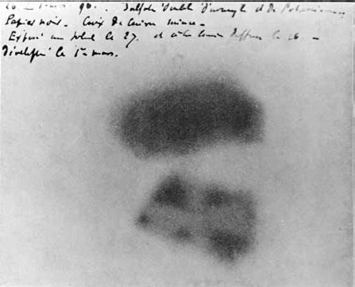Figure 57.2.1. Photographic plate made by Henri Becquerel. The plate shows marks where the plate was exposed to radiation from a uranium salt. Credits: Wikimedia Commons.

Further studies with non-phosphorescent uranium compounds, which also darkened the plates, convinced Becquerel that the rays responsible for darkening of the photographic plates in his experiments came from uranium itself and were a new phenomenon. After the discovery of radioactivity, Marie Skłodowska-Curie and her husband Pierre Curie found other materials that exhibited radioactivity. Other scientists at the time also worked on radioactivity and isolated radioactive elements. Henri Becquerel, Marie Skłodowska-Curie and Pierre Curie were awarded Nobel prize for Physics in 1903 for their work on radioactivity.

When Marie Curie separated uranium from pitchblende, a uranium ore, she found that the left-over material, which did not have any uranium, was also radioactive. She correctly interpreted that the left over part of the ore must contain some other radioactive element. It took several tons of pitchblende to isolate 100 mg of radium. In 1911 Marie Curie was awarded a second Nobel prize, this time in Chemistry, in recognition of her services to the advancement of chemistry by the discovery of the elements radium and polonium, by the isolation of radium and the study of the nature and compounds of this remarkable element.''

### Subsection57.2.1Alpha, beta, and gamma rays

The radiation from radioactive materials do not all consist of same particles. Experiments of Rutherford of the kind shown in Figure 57.2.2 showed that radiation from radioactive elements behaved differently when directed into a space with a magnetic field. The positively charged particles of alpha rays bend one way, the negatively charged particles of the negative beta rays bend the other way, and the uncharged particles of the gamma rays go undeviated. The three types of rays also differ in their ability to penetrate materials with the alpha rays being the least penetrating and gamma rays the most. Alpha rays can be stopped by a sheet of paper while it takes several cm of lead to shield from gamma rays. The beta rays can be stopped with a few mm thick aluminum.

The alpha rays are made up of the doubly charged helium nucleus, $^4_2$He, which are also called alpha particles. The beta rays consist of electrons or positrons. A positron has all the same properties as an electron, except that it has the opposite charge. We designate a positron with a plus sign, $e^{+}\text{,}$ and electron with a minus sign, $e^{-}\text{.}$ The electron rays are sometimes designated as $\beta^{-}$ rays and the positron rays as $\beta^{+}$ rays as in Figure 57.2.2. The gamma rays consist of photons which are uncharged and hence are not bent by the magnetic field.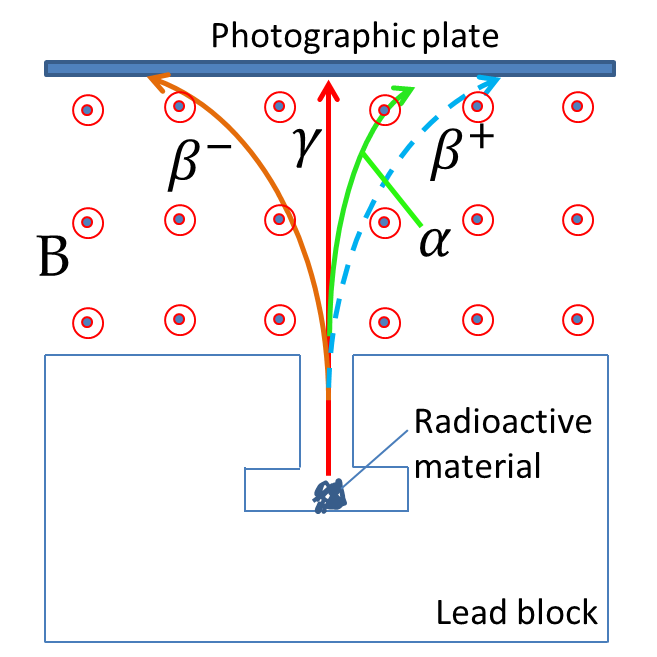Figure 57.2.2. Bending of alpha, beta, and gamma rays in a magnetic field. The $\alpha$ and $\beta^{-}$ rays bend in opposite directions in a magnetic field while $\gamma$ rays do not bend. The $\beta^{+}$ rays consist of positrons and bend in the same direction as the $\alpha$ rays.

Radioactive materials are often characterized by the type of ray they emit. Thus, we say that $^{238}_{92}$U is an alpha source, $^{23}_{11}$Na is a $\beta^{+}$ source, and Co is a gamma source.

### Subsection57.2.2The Decay Dynamics

The radioactive decay, whether it be $\alpha$-, $\beta$-, or $\gamma$-decay, or some other decay process consists of the transformation of a radioactive nucleus into some other nucleus and/or the change of the quantum state of the nucleus over time, e.g. from an excited nuclear state to the ground state of the nucleus. We will refer to both of these possibilities by a common phrase: decay. Since the decay event is a quantum mechanical process, the actual time it takes for a particular nucleus to decay is known only statistically. Therefore, we study radioactivity of large populations of radioactive nuclides statistically.

Let $N(t)$ represent the number of a radioactive nuclide in a given sample at time $t\text{.}$ Then, we find that the rate of decay, i.e. the rate at which $N(t)$ changes with time, is proportional to the number of radioactive nuclide at that time.

\begin{equation} \frac{dN}{dt} = -\lambda N.\label{eq-radioactivity-rate-equation}\tag{57.2.1} \end{equation}

The proportionality constant $\lambda$ is called the rate constant or decay constant. Let us bring $N$ to the left side of the equation and transfer $dt$ to the right to obtain fractional change in the number of radioactive nuclide during the interval $t$ to $t+dt$ to be

\begin{equation*} \frac{dN}{N} = -\lambda\: dt. \end{equation*}

Let $N_0$ be the number of radioactive atoms at $t=0\text{.}$ Integrating this equation from $t=0$ to $t=t$ means the integration on the left side will be from $N_0$ to $N$ and the integration on the right side will be from $t=0$ to $t=t$ giving

\begin{equation} \ln \frac{N(t)}{N_0}=-\lambda t\ \ \Longrightarrow\ \ N(t) = N_0 e^{-\lambda t}.\label{eq-radioactivity-rate-equation-sol}\tag{57.2.2} \end{equation}

This says that number of radioactive atoms in a sample decreases exponentially with time. In time $t = 1/\lambda\text{,}$ only $1/e$ of the original radioactive atoms would have remained in the original state.

An important concept associated with the decay rate is the half-life, $T_{1/2}\text{,}$ of a radioactive material. The half-life is defined to be the time it takes for half of the radioactive nuclei to have decayed into its products, or, equivalently, only half of the initial radioactive atoms are remaining in the sample. To find the half life we set $t=T_{1/2}$ and $N=\frac{1}{2}N_0$ in Eq. (57.2.2).

\begin{equation} \frac{N_0}{2} = N_0 e^{-\lambda T_{1/2}}. \label{eq-radioactivity-half-life-1}\tag{57.2.3} \end{equation}

We solve this equation for $T_{1/2}$ by dividing out $N_0$ and taking the natural logarithm of the two sides. This gives

\begin{equation} T_{1/2} = \frac{\ln 2}{\lambda} \approx \frac{0.693}{\lambda}.\label{eq-radioactivity-half-life-2}\tag{57.2.4} \end{equation}

Note that the half-life does not depend upon the number of atoms in the sample - it only depends on the rate of decay, $\lambda\text{.}$ For instance, if you have $10^{12}$ radioactive atoms in the sample at $t=0\text{,}$ then you will have $\frac{1}{2}\times 10^{12}$ radioactive atoms left at $t=T_{1/2}\text{,}$ and $\frac{1}{4}\times 10^{12}$ radioactive atoms left (which is half of $\frac{1}{2}\times 10^{12}$) at $t=2T_{1/2}\text{,}$ and so on. Note that $\lambda$ in Eq. (57.2.2) can be exchanged for half life $T_{1/2}$ as follows.

\begin{equation} N(t) = N_0 2^{- t /T_{1/2}} = N_0 \left( \frac{1}{2}\right)^{t /T_{1/2}}. \label{eq-radioactivity-rate-equation-sol-half}\tag{57.2.5} \end{equation}

Clearly, larger the decay rate, the more active the sample will be and smaller will be the half-life of the sample. The activity of a sample of a radioactive material is defined by the absolute value of the rate of change of $N\text{.}$

\begin{equation*} \textrm{Activity} = \left|\frac{dN}{dt}\right|. \end{equation*}

From Eq. (57.2.1) we can express this in terms of the number of radioactive atoms at the moment and the rate constant.

\begin{equation} \textrm{Activity} = \left|\frac{dN}{dt}\right| = \lambda N. \label{eq-nuclear-activity-first}\tag{57.2.6} \end{equation}

That is, a sample with more radioactive material will have a higher activity. The decay of one nucleus constitutes one event. Therefore, we define the unit of activity by number of decays in one second. This unit is also called 1 Becquerel (Bq).

\begin{equation*} 1\:\textrm{Bq} = 1\:\textrm{decay/second}. \end{equation*}

Radioactivity is often measured by a device called a Geiger counter in which radiation from each event enters a tube and ionizes a gas. The ionized gas is accelerated by high voltage which magnifies the signal for detection. In Geiger counter, each event gives one count and the activity is given by counts per second.

The activity is also given in terms of another unit called Curie (Ci). Originally, 1 Ci was defined by the activity of one gram of highly radioactive radium-226, but now by definition we state 1 Ci in terms of Bq.

\begin{equation*} 1\:\textrm{Ci} = 3.7\times 10^{10}\:\textrm{Bq}. \end{equation*}

It turns out that 1 Ci is too large a unit for most laboratory work. The milliCurie (mCi) and microCurie ($\mu$Ci) are in common use.

Eq. (57.2.6) can also be written in other forms by making use of other equations presented above.

\begin{equation} \textrm{Activity} = \left|\frac{dN}{dt}\right| = \left|\frac{dN}{dt}\right|_{t=0}\ e^{-\lambda t} = \left|\frac{dN}{dt}\right|_{t=0}\ \left(\frac{1}{2} \right)^{t/T_{1/2}}. \label{eq-nuclear-activity-various}\tag{57.2.7} \end{equation}

Tritium decays by beta decay according to the following equation.

\begin{equation*} ^{3}_{1}\textrm{H} \longrightarrow \ ^{3}_{1}\textrm{He} + e^{-1} + \bar\nu_e. \end{equation*}

The radioactivity of a chemical compound containing tritium was measured fifteen days apart. On the first day, the sample had an activity of 10,000 Bq and on the 16th day the activity was 9975. Determine the half life of tritium from this data.

Hint

Use Eq. (57.2.7).

$4,154\:\textrm{d}\text{.}$

Solution

We use Eq. (57.2.7) since the data is in activity. We will write the equation as

\begin{equation*} \textrm{Activity}[t]= \textrm{Activity[t=0]}\ \left(\frac{1}{2} \right)^{t/T_{1/2}}, \end{equation*}

where $t=0$ is the activity on the first day. Setting the $t=15$ days in this equation with the activity for the 16th day we get

\begin{equation*} 9975\: \textrm{Bq}= 10,000\: \textrm{Bq} \ \left(\frac{1}{2} \right)^{15\:\textrm{d}/T_{1/2}} \end{equation*}

Dividing both sides by 10,000 and taking the log of both sides we get

\begin{equation*} \frac{15\:\textrm{d}}{T_{1/2}}\:\log 2 = -\log 0.9975. \end{equation*}

Therefore,

\begin{equation*} T_{1/2} = \frac{15\:\textrm{d}\: \log 2}{-\log 0.9975} = 4,154\:\textrm{d} \end{equation*}

A radioactive material has a half life of 10 days. Initially, there is 128 $\mu$g of the material in a test tube. How much of the radioactive material is left after 50 days?

Hint

Use half-life.

$4\:\:\mu\textrm{g}\text{.}$

Solution

The duration $t$ is 5 half-lives. Therefore, the amount left will be

\begin{equation*} 128\:\mu\textrm{g} \times \left(\frac{1}{2} \right)^{5} = 4\:\:\mu\textrm{g}. \end{equation*}

The half life of the isotope $^{222}$Rn of radon is 3.8 days. Initially, there are $10^{12}$ atoms of $^{222}$Rn in the sample. How many atoms of $^{222}$Rn will remain after 30 days?

Hint

Use Eq. (57.2.5).

Solution

We use Eq. (57.2.5) to figure this out, where $t= 30$ days and $T_{1/2} = 3.8$ days.

\begin{equation*} N = 10^{12}\:\textrm{atoms} \times \left( \frac{1}{2}\right)^{(30\:\textrm{d}/3.8\:\textrm{d})} = 4.2 \times 10^9\:\textrm{atoms}. \end{equation*}

Radioactive dating refers to determining the age of a specimen by using the radioactivity of the sample. One application of radioactive dating is called carbon dating which is used to find the age of fossils of living organisms. The idea is based on the observation that the radioactive isotope $^{14}$C of carbon is generated by nuclear reaction with cosmic rays. It has been found that the level of $^{14}$C in the atmosphere has not changed much over a long period of time. So, we make an assumption that $^{14}$C in the atmosphere is a constant. Presently, there are

\begin{equation*} \textrm{Present value of } \frac{^{14}\textrm{C}}{^{12}\textrm{C}} = 1.2\times 10^{-12}. \end{equation*}

Plants and other living organisms exchange $^{14}$C and $^{12}$C from sources that are in equilibrium with the atmosphere. Thus, living organisms have a very steady ratio of the isotopes $^{14}$C to $^{12}$C in their bodies since a living organism exchanges carbon with the environment. Once an organism dies, the exchange of carbon with the environment stops. Since $^{12}$C is stable while $^{14}$C decays to $^{14}$N, the ratio of $^{14}$C to $^{12}$C in the dead organism decreases with time with the half life of 5,730 years, the half life of $^{14}$C. By assuming the $^{14}$C to $^{12}$C ratio in the organism when it died to be same as the ratio we find today in the living organisms, we can determine the age of the fossil. The carbon dating of ancient bristlecone pine trees have provided an independent check for the method to around 6,000 years with a margin of error of 600 or 700 years.

One g of pure carbon extracted from a living organism has activity of approximately 15 disintegration per minute. One milligram of carbon from a fossil shows an activity of $4\times 10^{-3}$ disintegration per minute. What is the age of the fossil?

Hint

See solution.

$10,900\:\textrm{y}\text{.}$

Solution

As described in the text, the activity in the living organism now will be assumed to be the activity in the fossil at the time $t=0$ when the organism died. For consistency in calculations, we will write all activities for 1 g of sample in units of per minute.

\begin{align*} \amp \left[ \frac{dN}{dt} \right]_{t=0} = -15\:\textrm{min}^{-1}\textrm{g}^{-1},\\ \amp \left[ \frac{dN}{dt} \right]_{t=t} = -4\times 10^{-3}\times 10^3 \:[\textrm{mg in g}] = -4\:\textrm{min}^{-1}\textrm{g}^{-1} \end{align*}

The half life of $^{14}$C in minutes is

\begin{equation*} T_{1/2} = 5730\:\textrm{y} \times 365.25 \times 24\times 60\: \textrm{min/y} = 3.01375\times 10^9\:\textrm{min}. \end{equation*}

This gives $\lambda$ in per min.

\begin{equation*} \lambda = \frac{0.693}{T_{1/2}} = \frac{0.693}{3.01375\times 10^9\:\textrm{min}} = 2.3\times 10^{-10}\:\textrm{min}^{-1}. \end{equation*}

We now write out the equations for the $t=0$ and $t=t$ and divide them to get rid of the common factors.

\begin{align*} \amp \left[ \frac{dN}{dt} \right]_{t=0} = -\lambda N_0,\\ \amp \left[ \frac{dN}{dt} \right]_{t=t} = -\lambda N_0\:e^{-\lambda t}, \end{align*}

where I have replaced $N$ on the right side in the equation by $N_0 e^{-\lambda t}\text{.}$ Dividing we get

\begin{equation*} e^{-\lambda t} = \left( \frac{dN}{dt} \right)_{t=t} \div \left( \frac{dN}{dt} \right)_{t=0}. \end{equation*}

Therefore, the time $t$ will be

\begin{equation*} t = \frac{1}{\lambda} \: \ln\left[ \left( \frac{dN}{dt} \right)_{t=0} \div \left( \frac{dN}{dt} \right)_{t=t} \right]. \end{equation*}

Now, we put in the numerical values to obtain

\begin{align*} t \amp = \frac{1}{2.3\times 10^{-10}\:\textrm{min}^{-1}} \: \ln\left[ 15\:\textrm{min}^{-1}\textrm{g}^{-1} \div 4\:\textrm{min}^{-1}\textrm{g}^{-1} \right]\\ \amp = 5.75\times 10^9 \textrm{min} = 10,900\:\textrm{y}. \end{align*}

### Subsection57.2.4Alpha Decay

In an alpha decay a nucleus $^A_Z$X spontaneously loses two protons and two neutrons when it emits a $^4_2$He particle and transforms to another nucleus Y with $Z$ two less than that of X and $A$ four less than that of X. We can write this nuclear transformation as follows.

\begin{equation} ^A_Z\textrm{X} \longrightarrow\ ^{A-4}_{Z-2}\textrm{Y} + ^4_2\textrm{He}. \label{eq-alpha-decay-nuc-rx}\tag{57.2.8} \end{equation}

The original nucleus $X$ is called the parent nucleus and the product nucleus Y is called the daughter nucleus. When writing nuclear reactions such as Eq. (57.2.8) we make sure that the two sides of the arrow have:

1. the same $A\text{,}$ and,
2. the same electric charge.

In this reaction if X was a neutral atom then Y will have to have charge $-2e$ since $\alpha$ particle has a charge of $+2e\text{.}$ One could display the electric charge information in Eq. (57.2.8) and write it as follows.

\begin{equation} ^A_Z\textrm{X}^{0} \longrightarrow\ ^{A-4}_{Z-2}\textrm{Y}^{-2} + ^4_2\textrm{He}^{+2}. \label{eq-alpha-decay-nuc-rx-2}\tag{57.2.9} \end{equation}

However, this notation will be too cumbersome and the convention is to omit charges on nulcides and alpha particles and keep the charge information implicit.

There are numerous examples of $\alpha$ decay. Each alpha decay is characterized by its half-life. For instance, the $\alpha$ source used by Rutherford in his scattering experiment that led to the discovery of nucleus was purified radium, $^{226}_{\ 88}$Ra, which transforms into radon with alpha decay,

\begin{equation*} ^{226}_{\ 88}\textrm{Ra} \longrightarrow\ ^{222}_{\ 86}\textrm{Rn} + ^4_2\textrm{He} \ \ \ \ \ \ (T_{1/2} = 1600\:\textrm{yr}) \end{equation*}

Polonium-210 blamed for the death of the Russian spy Alexander Litvinenko in 2006 is an alpha emitter with the following reaction.

\begin{equation*} ^{210}_{\ 84}\textrm{Po} \longrightarrow\ ^{222}_{\ 82}\textrm{Pb} + ^4_2\textrm{He} \ \ \ \ \ \ (T_{1/2} = 138\:\textrm{days}) \end{equation*}

The element polonium was originally discovered in 1897 by Marie Curie who named the element after her country of birth, Poland. Many other nuclides decay by alpha decay process.

When a parent nuclide X decays we find that the rest mass of the parent nuclide X is larger than the sum of the rest masses of the daughter nuclide Y and the $\alpha$ particle.

\begin{equation*} m_{\textrm{X}} > m_{\textrm{Y}} + m_{\alpha}. \end{equation*}

Since energy is conserved in the decay, the difference in the rest energies of the parent and the products shows up in the kinetic energies of the daughter nucleus and the alpha particle. The difference in the the rest energies of the starting nuclide and the products is called the disintegration energy which is usually denoted by the letter $Q$ [do not confuse this with the same symbol for charge].

\begin{equation*} Q = m_{\textrm{X}} c^2 - \left( m_{\textrm{Y}} c^2+ m_{\alpha} c^2\right). \end{equation*}

In alpha decay of large nuclei, most of this energy ends up in the kinetic energy of the alpha particles as we can show by applying conservation of energy and conservation of momentum to the alpha decay reaction as we will study below. For alpha decay $Q>0\text{.}$ If $Q \lt 0$ for some hypothetical process, then the process will not take place spontaneously, one would need to put energy into the process to make it happen, if at all.

Consider a nuclide X at rest decay into a nuclide Y and an alpha particle $\alpha\text{.}$ Let $K_\textrm{Y}$ and $K_\alpha$ be the kinetic energies of Y and $\alpha$ particle. Let $p_\textrm{Y}$ and $p_\alpha$ be the momenta of Y and $\alpha$ particle, which would be in the opposite directions for the momentum to add up to zero, which is the momentum of X. The conservation of energy and momentum gives the following two equations.

\begin{align} \amp \textrm{Conservation of Energy:}\ \ \ \left( m_{\textrm{Y}} c^2+ m_{\alpha} c^2\right) +K_\textrm{Y} + K_\alpha = m_{\textrm{X}} c^2. \label{eq-alpha-enery-cons-1}\tag{57.2.10}\\ \amp \textrm{Conservation of Momentum:}\ \ \ p_\textrm{Y} - p_\alpha = 0.\label{eq-alpha-mom-cons-1}\tag{57.2.11} \end{align}

In the second equation, we can write the momenta in terms of the kinetic energies. With the assumption that the speeds are non-relativistic we will have

\begin{equation*} K = \frac{p^2}{2m},\ \ p = \sqrt{2m K}. \end{equation*}

Rewriting Eq. (57.2.11) in terms of kinetic energies we get

\begin{equation} m_\textrm{Y} K_\textrm{Y} = m_\alpha K_\alpha.\label{eq-alpha-mom-cons-2}\tag{57.2.12} \end{equation}

We also rewrite Eq. (57.2.10) using $Q$ for the disintegration energy part. We get

\begin{equation} K_\textrm{Y} + K_\alpha = Q. \label{eq-alpha-enery-cons-2}\tag{57.2.13} \end{equation}

We can now solve Eqs. (57.2.12) and (57.2.13) for $K_\alpha$ and $K_\textrm{Y}\text{.}$

\begin{align*} \amp K_\alpha = \frac{m_{\textrm{Y}}}{m_{\textrm{Y}} + m_{\alpha}}\: Q.\\ \amp K_\textrm{Y} = \frac{m_{\alpha}}{m_{\textrm{Y}} + m_{\alpha}}\: Q. \end{align*}

If $m_\alpha \ll m_{\textrm{Y}}\text{,}$ which is the case with heavy nuclides, we will have approximately

\begin{equation} \left. \begin{array}{l} K_\alpha \approx Q,\\ K_\textrm{Y} \approx \frac{m_{\alpha}}{m_{\textrm{Y}} }\: Q \end{array} \right\} \ \ (m_\alpha \ll m_{\textrm{Y}}).\label{eq-alpha-decay-kinetic-energies}\tag{57.2.14} \end{equation}

(a) Calculate the energy released in the alpha decay of $^{238}_{\ 92}$U into $^{234}_{\ 90}$Th.

\begin{equation*} ^{238}_{\ 92}\textrm{U} \longrightarrow\ ^{234}_{\ 90}\textrm{Th} \ +\ ^{4}_{2}\textrm{He}. \end{equation*}

The masses of nuclides are

\begin{equation*} m[^{238}_{\ 92}\textrm{U}] = 238.050 788 26 \:\textrm{u};\ \ m[^{234}_{\ 90}\textrm{Th}] = 234.043 601 \:\textrm{u}; \ \ m[^{4}_{2}\textrm{He}] = 4.002 054 76 \:\textrm{u}; \end{equation*}

(b) What are the kinetic energies of the alpha particle and Th-239?

Hint

(a) $4.78\:\textrm{MeV}\text{,}$ (b) $0.082\:\textrm{MeV}\text{.}$

Solution

The $Q$ is given by the difference in the rest energies of the starting nuclide and ending nuclides.

\begin{equation*} Q = m[^{238}_{\ 92}\textrm{U}] c^2 - \left( m[^{234}_{\ 90}\textrm{Th}] + m[^{4}_{2}\textrm{He}]\right) c^2. \end{equation*}

The masses are given in the unit u, when when multiplied by $c^2$ will be

\begin{equation*} 1\: \textrm{u} \times c^2 = 932\:\textrm{MeV}. \end{equation*}

Therefore we can get the $Q$ directly in terms of MeV by

\begin{equation*} Q = \left[ 238.050 788 26 - 234.043 601 - 4.002 054 76\right]\:\textrm{u}\times 932\:\frac{\textrm{MeV}}{\textrm{u}} = 4.78\:\textrm{MeV}. \end{equation*}

In these calculations there are many significant figures. It is important to keep these figures in the calculation since often the multipliers are large.

(b) From Eq. (57.2.14) we obtain the required kinetic energies

\begin{equation*} K_\alpha \approx Q = 4.78\:\textrm{MeV}. \end{equation*}

Therefore,

\begin{align*} K_\textrm{Y} \amp \approx \frac{m_{\alpha}}{m_{\textrm{Y}} }\: Q\\ \amp = \frac{4.002 054 76\:\textrm{u}}{234.043 601\:\textrm{u}}\times 4.78\:\textrm{MeV} = 0.082\:\textrm{MeV}. \end{align*}

### Subsection57.2.5Beta Decay

The emission of electrons and positrons from a nucleus are usually called beta decay. This does not mean that electrons and positrons were inside the nucleus before the decay. Rather, they are formed during the process of decay accompanied by the conversion of neutron to proton or of proton to neutron by the following fundamental nuclear reactions similar to when an excited atom gives off a photon, we do not claim that the photon was inside the atom.

\begin{align} \amp n \longrightarrow\ p^{+} + e^{-} + \bar{\nu}_e,\ \ \ (\textrm{negative beta decay},\beta^{-}) \label{eq-beta-decay-1}\tag{57.2.15}\\ \amp p^{+} \longrightarrow\ n + e^{+} + \nu_e, \ \ \ (\textrm{positive beta decay},\beta^{+}) \label{eq-beta-decay-2}\tag{57.2.16} \end{align}

where $\nu_e$ and $\bar{\nu}_e$ are neutrino and antineutrino (more specifically electron-neutrino and anti-electron-neutrino) respectively. The mass of a neutrino is much less than even the mass of an electron, which is the lightest particle with non-zero mass whose mass is known with some accuracy. We will assume the mass of a neutrino to be zero and its speed to be equal to the speed of light:

\begin{equation*} m_{\nu} = 0,\ \ v_{\nu} = c. \end{equation*}

Therefor the energy and momentum of a neutrino will have the same relation as for photons, the particle of light.

\begin{equation*} E_{\nu} = p_{\nu} c. \end{equation*}

The reactions in Eq. (57.2.15)$-$(57.2.16) occurring in nuclei of atoms undergoing a beta decay.

\begin{align} \amp ^{A}_{Z}\textrm{X} \longrightarrow\ ^{A}_{Z+1}\textrm{Y} + e^{-} + \bar{\nu}_e, \ \ \ (\textrm{negative beta decay},\beta^{-})\label{eq-beta-decay-1a}\tag{57.2.17}\\ \amp ^{A}_{Z}\textrm{X}\longrightarrow\ ^{A}_{Z-1}\textrm{Y} + e^{+} + \nu_e, \ \ \ (\textrm{positive beta decay},\beta^{+}) \label{eq-beta-decay-2a}\tag{57.2.18} \end{align}

Some examples of these beta decays are shown in Table 57.2.9 along with the disintegration energy and the half-life time for each. As in the table, it is common practice to omit the $Z$ from the symbol of the nuclide since it is understood from the symbol of the element itslef.

\begin{equation*} ^ {A}_{Z}\textrm{X}\ \equiv\ \ ^{A}\textrm{X}. \end{equation*}

The disintegration energy $Q$ of a beta decay is defined in a similar way as we have done for the $\alpha$ decay by the difference in rest energies of the starting nuclide and the ending products.

\begin{equation*} Q = m_{\textrm{X}} c^2 - \left(m_{\textrm{Y}} c^2 + m_e c^2 + m_\nu c^2 \right). \end{equation*}

Since $m_\nu =0$ we get

\begin{equation*} Q = m_{\textrm{X}} c^2 - \left(m_{\textrm{Y}} + m_e \right) c^2. \end{equation*}

Now, note that we can add the masses of $X$ electrons to $m_{\textrm{X}}$ and $X+1$ electrons to $m_{\textrm{Y}}$ and convert this equation to the masses of the atoms, which we denote by the capital letter $M\text{.}$

\begin{equation*} Q =\left( M_{\textrm{X}} - M_{\textrm{Y}} \right) c^2. \end{equation*}

For instance, the $Q$ value of the $^{14}_{\ 6}$C beta decay is

\begin{align*} Q \amp = \left(m[^{14}_{ }C] - m[^{14}_{ }N]\right) c^2\\ \amp = \left( 14.00324989 \: \textrm{u} - 14.003074004\: \textrm{u}\right) c^2 = 0.164\:\textrm{MeV}. \end{align*}

Unlike the alpha decay, where the disintegration energy ends up as the kinetic energy of alpha particles, in the beta decay, the disintegration energy is shared by electron (or positron) and neutrino with a certain probability of a portion of the energy as the kinetic energy of the electron and the rest in the neutrino.

\begin{equation*} K_{e} + E_\nu \approx Q=\left( M_{\textrm{X}} - M_{\textrm{Y}} \right) c^2. \end{equation*}

Therefore, electrons (or positrons) coming out of a sample of beta-emitting sample have various energies upto a maximum, corresponding to the decay with $E_\nu =0\text{.}$

\begin{equation*} K_{e}^{\textrm{max}} = Q. \end{equation*}

Figure 57.2.10 shows the relative number of electrons with various kinetic energies in the beta decay spectrum of $^{14}$C. The maximum kinetic energy is equal to 0.156 MeV which is equal to the disintegration energy. In the decay of each nucleus the sum of the energy carried by the electron and the neutrino is equal tot 0.156 MeV although the energies of electron and neutrino emitted from the decay of different nuclei may be different.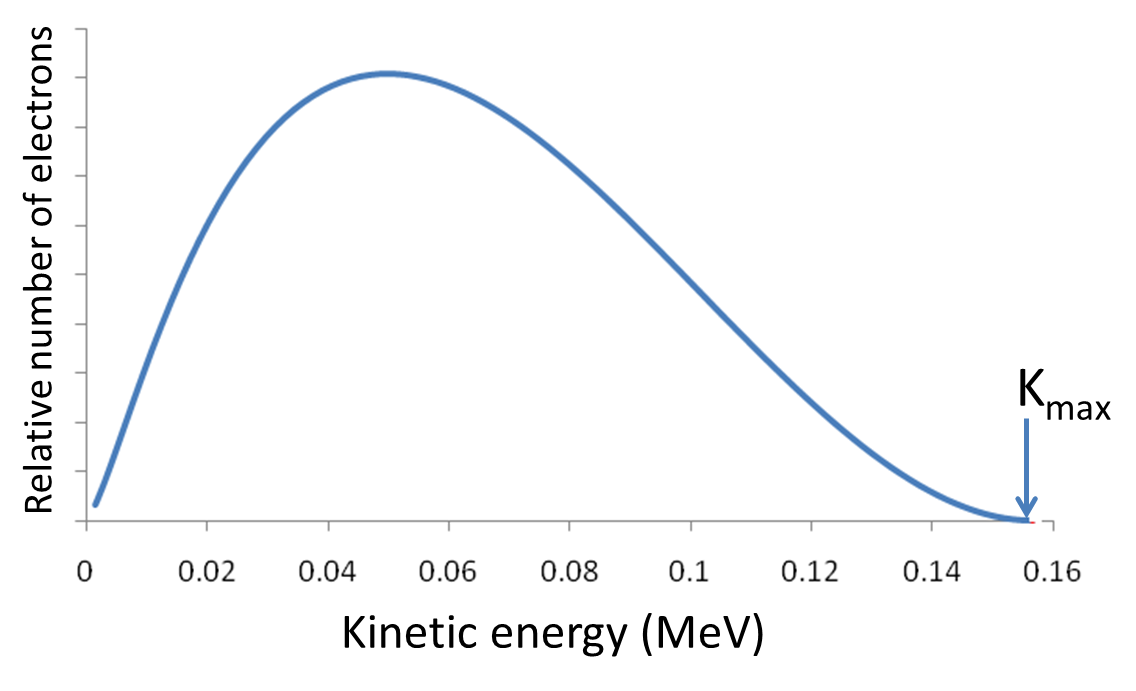Figure 57.2.10. The distribution of kinetic energy among electrons in the beta decay of $^{14}$C. For each electron emitted a neutrino is also emitted that carries the energy such that the sum of the energy of the neutrino and the kinetic energy of the electron equals the dissociation energy, which is 0.156 MeV for the $^{14}$C to $^{14}$N decay.

#### Subsubsection57.2.5.1Electron Capture

A related process to the process of emission of electrons and positrons is the capture of an electron from the orbit closest to the nucleus. Such an event usually occurs when the nucleus captures an electron from the K or L shell of the atom. The capture of an electron leads to a nuclear reaction that converts a proton into a neutron and a neutrino is released in the process.

\begin{equation} p^{+} + e^{-}[\textrm{orbital}] \longrightarrow\ n + \nu_e.\ \ \ (\textrm{Orbital electron capture},\mathcal{E}) \label{eq-beta-decay-3}\tag{57.2.19} \end{equation}

This reaction, called electron capture, is hard to detect directly since the released products are the daughter nucleus and the neutrino. However, as a result of the capture of the inner-shell electron, an electron from a higher enegy state falls into the inner shell to fill the empty level, which causes X rays to be emitted, which can be detected without too much difficulty. The increase in the number of daughter nuclides will also be a signal for this reaction mode. The reaction in Eq. (57.2.19) occurring in nuclei of atoms undergoing a beta decay can be written as

\begin{align*} \amp ^{A}_{Z}X + e^{-}[\textrm{orbital}] \longrightarrow\ ^{A}_{Z-1}Y + \nu_e.\ \ \ (\textrm{Orbital electron capture},\mathcal{E}) \end{align*}

The $Q$ of this reaction must take into account the binding energy pf the captured electron, which can be considerable.

\begin{equation} Q = \left( m_X - m_Y \right) c^2 - E_{K}, \label{eq-nuclear-q-electron-capture}\tag{57.2.20} \end{equation}

where $E_{K}$ is the binding energy of the captured electron. The mass of the electron on the left side of the reaction is already included in the mass of the nuclide $m_X\text{.}$ The following transformations exemplify electron capture process.

 $^{41}_{ }\text{Ca} + e^{-} \longrightarrow\ ^{41}_{ }\text{K} + \nu_e$ $(Q =0.43 \text{ MeV})$ $( T_{1/2} = 1.0\times 10^5 \text{ yr})$ $^{49}_{ }\text{V} + e^{-} \longrightarrow\ ^{49}_{ }\text{Ti} + \nu_e$ $(Q = 0.620\text{ MeV})$ $(T_{1/2} = 337 \text{ days})$ $^{15}_{ }\text{O} + e^{-} \longrightarrow\ ^{15}_{ }\text{N} + \nu_e$ $(Q = 2.75 \text{ MeV})$ $( T_{1/2} = 1.22 \text{ sec})$

Some nuclides undergo all three types of beta decay. For instance, $^{40}$K is just such a nuclide. About 89.28\% of the time, it undegoes $\beta^{-}$ decay to $^{40}$Ca, 10.72\% of the time undergoes electron capture $\mathcal{E}$ to an excited state of $^{40}$Ar (which de-excites and gives of gamma rays), and about 0.001\% of the time $\beta^{-}$ decay to $^{40}$Ar.

Compute the $Q$ value of K-shell capture

\begin{equation*} ^{15}_{8}\textrm{O} + e^{-} \longrightarrow\ ^{15}_{7}\textrm{N} + \bar{\nu}_e. \end{equation*}

The binding energy of K-shell electron, $E_K = 0.543$ keV. Masses of the isotopes are: $^{15}_{8}$O 15.003065 u, $^{15}_{7}$N 15.000108 u.

Hint

Use Eq. (57.2.20)

$2.75388\:\textrm{MeV}\text{.}$

Solution

From Eq. (57.2.20) we get

\begin{equation*} Q = \left( 15.003065\:\textrm{u} - 15.000108\:\textrm{u}\right) c^2\times 931.494\frac{\textrm{MeV}}{c^2} - 0.000543\:\textrm{MeV} = 2.75388\:\textrm{MeV} . \end{equation*}

### Subsection57.2.6Gamma Decay

A Gamma decay occurs when a nucleus makes quantum transition from a nuclear excited state to a state of lower energy by emitting a high energy photon, called gamma particles (which are high energy photons) in this context. We usually represent the excited state by placing a star on the symbol. Since gamma decay involves the emission of a photon only, which has no charge and no mass, the $A$ and $Z$ of the nucleus do not change.

\begin{equation*} ^{A}_{Z}\textrm{X}^* = ^{A}_{Z}\textrm{X} + \gamma. \end{equation*}

It is common for a nucleus to end up in an excited state as a result of the alpha or beta decay and then undergo the gamma decay. For instance, when $^{40}$K undergoes an electron capture, the daughter nucleus $^{40}$Ar is actually in an excited state, $^{40}$Ar$^*\text{.}$

\begin{equation*} ^{40}_{ }\textrm{K} + e^{-} \longrightarrow\ ^{40}_{ }\textrm{Ar}^{*} + \nu_e. \end{equation*}

The excited $^{40}_{ }\textrm{Ar}^{*}$ undergoes nuclear transition to the lower energy state and releases 1.460 MeV of energy in a photon.

\begin{equation*} ^{40}_{ }\textrm{Ar}^{*} \longrightarrow\ ^{40}_{ }\textrm{Ar} + \gamma. \end{equation*}

The energy diagram in Figure 57.2.12 represents the beta and gamma decays for the entire process. The wavelength of this photon is very small as can be seen by

\begin{equation*} \frac{hc}{\lambda} = E\ \ \Longrightarrow\ \ \lambda = \frac{hc}{E} = \frac{1.23984193\:\mu\textrm{m}.\textrm{eV}}{1.460\;\textrm{MeV}} = 0.85\:\textrm{pm}. \end{equation*}

For comparison, the X ray from a Molybdenum target is 71 pm.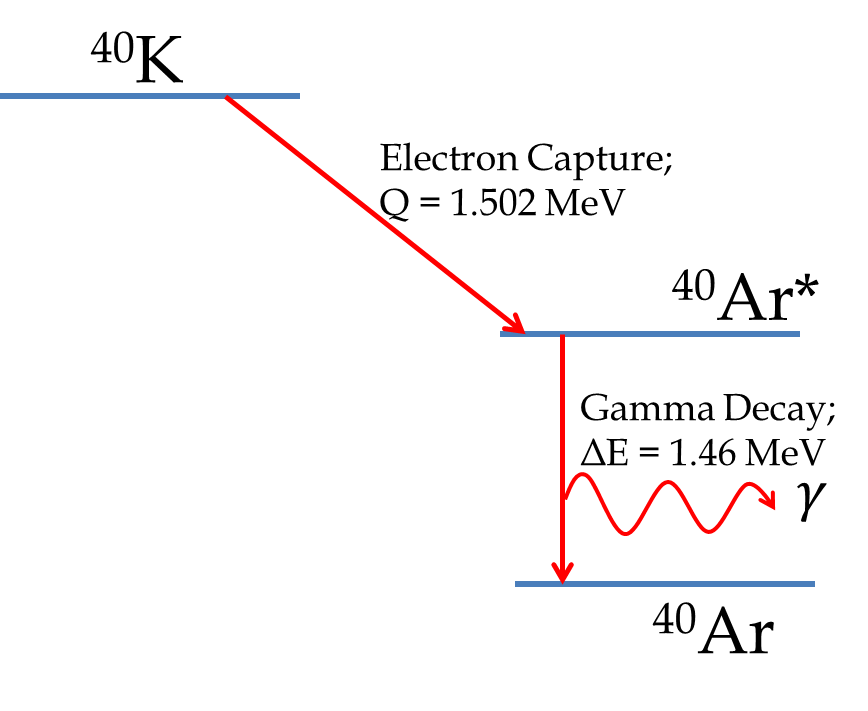Figure 57.2.12. The Decay scheme of $^{40}$K through the electron capture and then subsequent gamma emission from the excited nucleus of $^{40}$Ar.

A common source of gamma rays is an artificially produced cobalt nuclide $^{60}$Co. The decay scheme of $^{60}$Co is shown in Figure 57.2.13. $^{60}$Co decays by beta decay by two modes into two different excited nuclear states of $^{60}$Ni. Each of these excited states then give off photons for eventually relaxation to the ground state. Therefore, here we get two different energy gamma particles from the inter-nuclear transitions of $^{60}$Ni.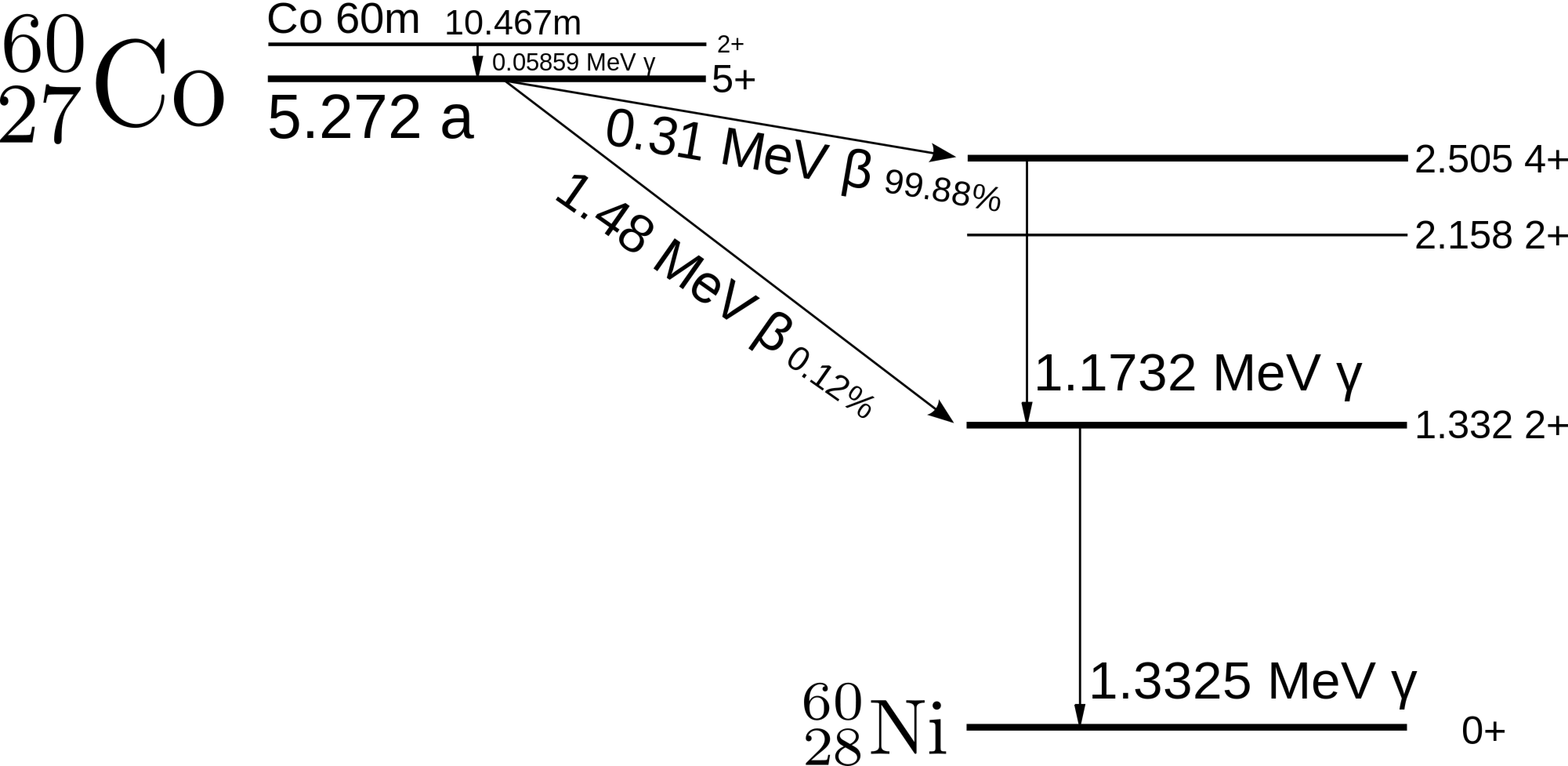Figure 57.2.13. The Decay scheme of $^{60}$Co through the electron capture and then subsequent gamma emissions from the excited nuclear states of $^{60}$Ni. The metastable state of $^{60}$Co, labeled Co60m is also shown. Credits: Wikicommons.

Radioactivity on Earth occurs in naturally occurring radionuclides as well as radioactive nuclides produced in nuclear reactions in laboratory. The radioactivity of $^{60}$Co discussed above is an example of the latter since $^{60}$Co is produced by nucelar reaction in which a neutron reacts with the nucleus of $^{59}$Co.

\begin{equation*} ^{59}\textrm{Co} + \textrm{n} =\ ^{60}\textrm{Co}, \end{equation*}

which decays by various channels and has a half life of 5.2714 years. The naturaly occuring radionuclides are of two types

1. Radionuclides that are either produced by nuclear reactions with cosmic rays. Carbon-14 is an example of a nuclide which is produced by the nuclear reaction of a neutron with the nucleus of $^{14}$N in atmosphere.
\begin{equation*} ^{14}\textrm{N} + \textrm{n} \longrightarrow\ ^{14}\textrm{C} + \textrm{p}^{+}. \end{equation*}
2. Long half-life radionuclides present at the time of the formation of the Earth approximately $4.54\times 10^9$ years ago. They are $^{238}_{\ 92}$U, $^{235}_{\ 92}$U, and $^{232}_{\ 90}$Th with half lives of $4.47\times 10^9$ years, $7.04\times 10^8$ years, and $1.41\times 10^{10}$ years, respectively.

These long-lived radionuclides decay into daughter nuclides that are themselves radioactive, whose daughters are also radioactive, and so on, till the final daughter is a stable isotope. The final product of the decay series starting with $^{238}_{\ 92}$U, $^{235}_{\ 92}$U, and $^{232}_{\ 90}$Th are three stable isotopes of lead, $^{206}_{\ 82}$Pb, $^{207}_{\ 82}$Pb, and $^{209}_{\ 82}$Pb, respectively. The uranium $^{238}$U series is shown in Figure 57.2.14.

There is also a radioactive series that can be started with the radionuclide $^{237}_{\ 93}$Np, but since the half life $^{237}_{\ 93}$Np is only $2.14\times 10^6$ years, none of the original $^{237}_{\ 93}$Np is left to continue the fourth series. The $^{237}_{\ 93}$Np ends at an isotope of Bismuth, $^{209}_{\ 83}$Bi rather than at the lead.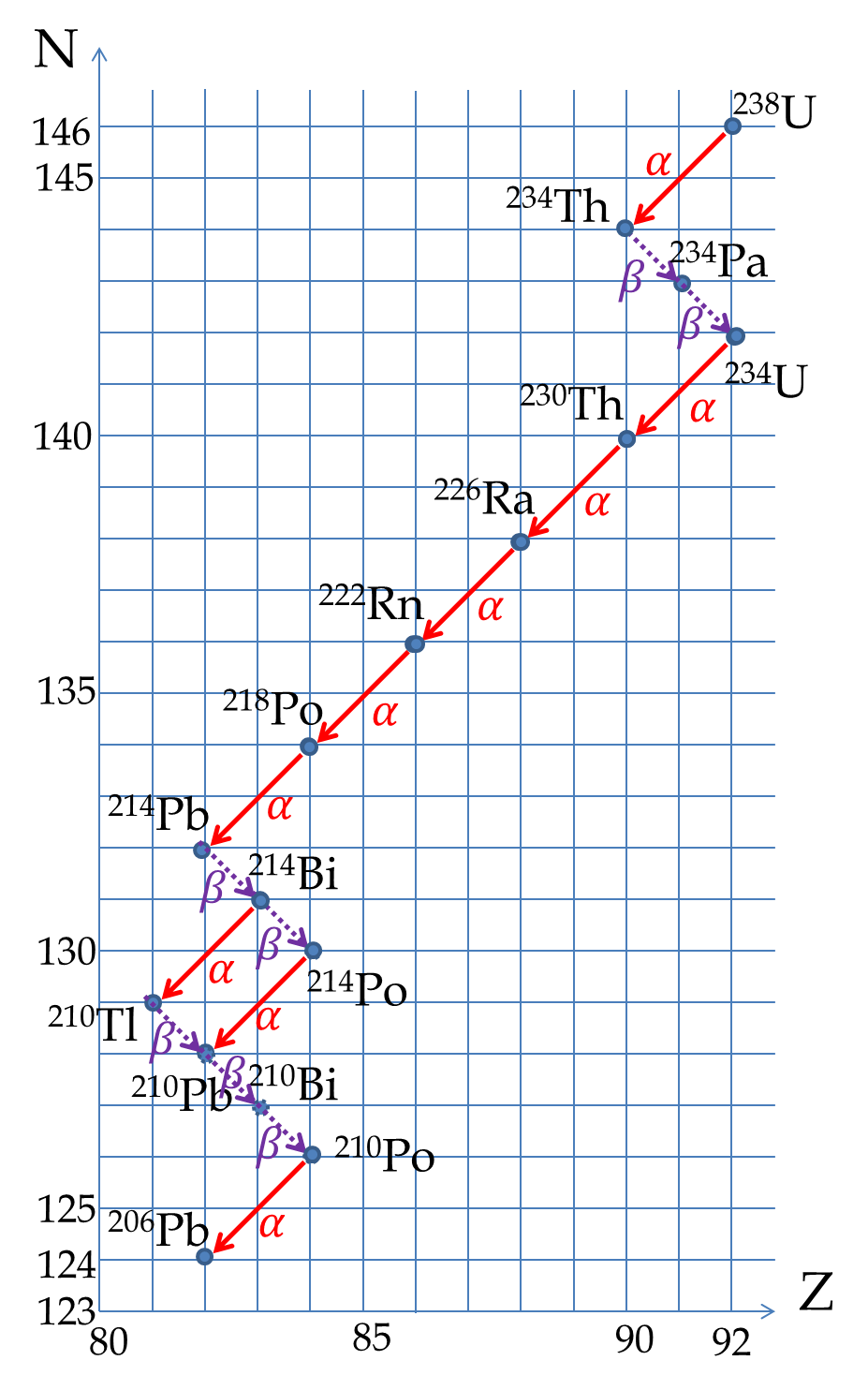Figure 57.2.14. The uranium $^{238}$U nuclear decay series ending at $^{206}$Pb. The first step in the series is an alpha decay with half life of $4.7\times 10^{9}$ years indicating that much of $^{238}$U at the time of formation of Earth are still around.
TODO:

\​begin{exercise} The isotope $^{32}$P decays by negative beta decay with a half life of 14.28 d. (a) If there was $1.0\time 10^{10}$ atoms of $^{32}$P in the sample at $t=0$, how many will be left in a 142.8 days? (b) How many will be left in 365 days? \end{exercise}

\​begin{exercise} An unknown radioactive material is decaying at a rate of 5,000 counts per second. You wait two weeks and measure the activity and find that the counts now is 2,500 counts per second. What is is half life of the material? \end{exercise}

\​begin{exercise} An unknown radioactive material is decaying at a rate of 50,000 counts per min. You wait two weeks and measure the activity and find that the counts now is 1,150 counts per minute. What is is half life of the material? \end{exercise}

\​begin{exercise} (a) Compute the number of molecules of potassium chloride KCl in 1 g of KCl. (b) Suppose 1\% of potassium atoms are in isotope $^{40}$K. How many $^{40}$K atoms will be in 1 g of KCl? (c) Normally KCl has a slight radioactivity. Supposing the radioactivity comes from $^{40}$K atoms in KCl, what will be the activity in decays per second of 1 g KCl if the half life of $^{40}$K is $1.3 \times 10^9$ y? \end{exercise}

\​begin{exercise} In Fukushima, Japan, 13,500 TBq (Tera Becquerel) of Cs-134 was released. Cs-134 is a beta emitter with a half life of 30.2 y. What is the total mass of Cs-134 released? \end{exercise}

\​begin{exercise} Complete the following decay reactions with appropriate symbols for the atomic species and other particles. \​begin{enumerate}[(a)] \item $^{154}_{64}\textrm{Gd} \longrightarrow\ ^{4}_{2}\textrm{He} \ +\ ?$ \item $^{238}_{92}\textrm{U} \longrightarrow\ ^{4}_{2}\textrm{He}\ +\ ?$ \item $^{231}_{91}\textrm{Pa} \longrightarrow\ ^{4}_{2}\textrm{He} \ +\ ?$ \item $\ \ ?\ \ \ \longrightarrow\ ^{4}_{2}\textrm{He} +\ ^{181}_{77}\textrm{Ir}$. \item $\ \ ?\ \ \ \longrightarrow\ ^{4}_{2}\textrm{He} +\ ^{228}_{88}\textrm{Ra}$. \end{enumerate} \end{exercise}

\​begin{exercise} Complete the following beta decay reactions with appropriate symbols for the atomic species and other particles. \​begin{enumerate}[(a)] \item $^{131}_{51}\textrm{I} \longrightarrow\ ?$ \ \ \ \ (beta minus) \item $^{247}_{95}\textrm{Am} \longrightarrow\ ?$ \ \ \ \ (beta minus) \item $^{40}_{19}\textrm{K} \longrightarrow\ ?$ \ \ \ \ (beta plus) \item $^{23}_{12}\textrm{Mg} \longrightarrow\ ?$ \ \ \ \ (beta plus) \end{enumerate} \end{exercise}

\​begin{exercise} Find the amount of energy released when $^{238}$U decays into $^234$Th by an alpha decay process. Data: M(U238) = 238.0507847 u, M(Th234) = 234.036596 u. \end{exercise}

\​begin{exercise} An unbound neutron decays rapidly by beta decay. Find the maximum energy of the electron in the beta decay of a neutron. \end{exercise}

\​begin{exercise} The isotope $^{223}$Ra has a potential use in cancer therapy. It decays by alpha decay into radon. (a) Find the $Q$ of the reaction. (b) If an atom of Ra at rest decays, what is the kinetic energy of the alpha particle that comes out? (c) What is the kinetic energy of the radon atom that forms? Data: M(Ra223) = 223.018497 u, M(Rn219) = 219.00948 u. \end{exercise}

\​begin{exercise} The isotope $^{137}$Cs is a beta minus emitter. (a) Write the equation of the beta decay. (b) Determine the $Q$ of the reaction? (c) What is the maximum kinetic energy of the electron released? (d) What is the maximum energy of the antineutrino released? \end{exercise}

\​begin{exercise} The half lives of the two isotopes of Uranium are: ($^{235}$ U, $7.04\times 10^{8}$ y), ($^{238}$ U, $4.47\times 10^{9}$ y). The current abundances of the two isotopes, $^{235}$ U and $^{238}$ U in natural deposits are 0.7\% and 99.3\% respectively. What were the corresponding abundances when Earth was formed 4.54 billion years ago? \end{exercise}

\​begin{exercise} Prove $N(t) = N_0 2^{-t/T_{1/2}}$. \end{exercise}